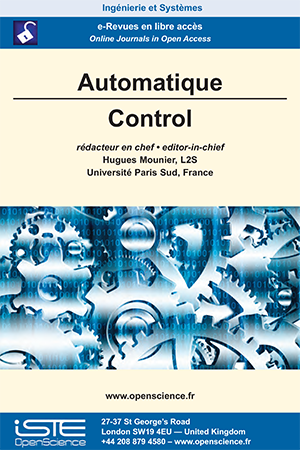# Vol 1 - Issue 1

## List of Articles

Recursive identification in transmittance of under‐operated mechanical system (applied to inversed pendulum)

A new approach of identifying mechanical system under-operated is presented in this article. The basic idea
is to spread the extensive least-square algorithm and the Stieglitz and McBride algorithm to the case of under-operated
systems. The considered models are OE and ARMAX type. The comparative evaluation through simulation using
numerical example shows clearly the quality of the identification using this approach compared to the result obtained by
the non-recursive linear least-squared methods. The use of the OE and ARMAX models has made the improvement of
the asymptotic convergence of these algorithms possible.

Discussing an intriguing result on model-free control

An elementary mathematical example proves, thanks to the Routh-Hurwitz criterion, a result that is intriguing with respect to today’s practical understanding of model-free control, i.e., an “intelligent” proportional controller (iP) may turn to be more difficult to tune than an intelligent proportional-derivative one (iPD). The vast superiority of iPDs when compared to classic PIDs is shown via computer simulations. The introduction as well as the conclusion analyse model-free control in the light of recent advances.

A Study of non linear state estimation based on invariant observers

This article presents a study of non linear state estimation based on invariant preserving observer : l’IUKF (Invariant Unscented Kalman Filter ). Several research works on nonlinear invariant observers have been led and provide a geometrical-based constructive method for designing filters such as the IEKF (Invariant Extended Kalman Filter ) wihle preserving the physical properties and systems symmetries. The developed IUKF estimator suggests a systematic approach to determine all the symmetry-preserving correction terms, without requiring any linearization of differential
equations or compatibility condition. The IEKF and IUKF algorithms are compared on academic case of tilt sensor system. The developed IUKF is then used with an aided Inertial Navigation System (INS). The results show promising perpectives and demonstrate that nonlinear state estimation converges on a much bigger set of trajectories than for more traditional approaches.2017

Volume 17- 1

Issue 1

2018

Volume 18- 2

Issue 1

2021

Volume 21- 3

Issue 1